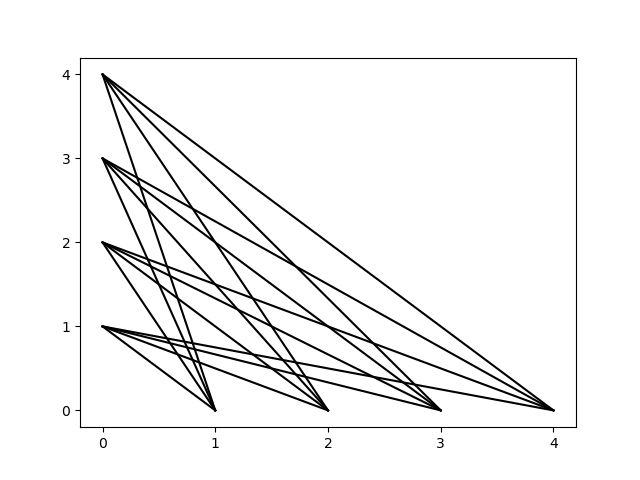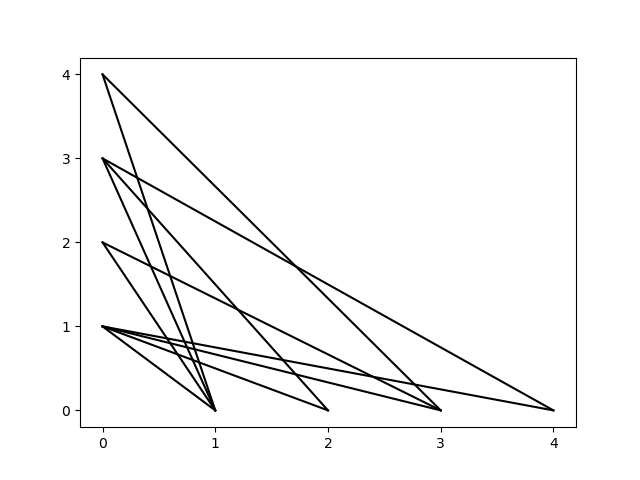### C - Straight Lines

##### Languages: C, C++, Java, Python, ... (details)

You have every point with positive integer coordinates not greater than $N$ over the $x$ and $y$ axis available and you want to draw as many straight lines as you can, with the condition that every line must pass through one of those points on each axis and there are no parallel lines. How many lines can you draw? How many ways can you draw these lines to achieve this maximum?These two images correspond to sample case where $N = 4$:

• The image on the left shows all the lines you could draw if there were no restriction over parallel lines.
• The image on the right shows one way to draw $11$ lines where no parallel lines are allowed.

#### Input

A line with an integer $N$ ($1 \le N \le 10^9$).

#### Output

A line with two integers $c$ and $w$, representing the maximun number of lines you can draw and the number of ways, modulo prime $998244353$, to achieve that result.

Input
1
Output
1 1
Input
4
Output
11 16